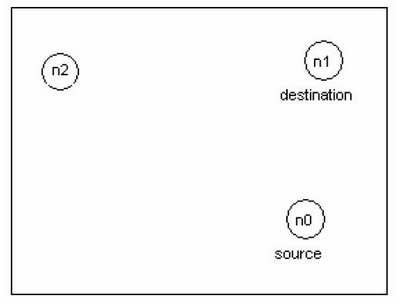## Mar 16, 2006

### Exercise - Simulate Ad Hoc Network

General

The objective of this assignment is to get familiar with the network simulator tool (ns-2) and mobile wireless network environment. This study about ad hoc wireless network will be carried out by simulations using the ns-2 tool.
These simulations will be run using Sun workstations of the Birdland (Lintula). The results of the work will be reported in writing.

In this document there are first some are conceptual definitions, instructions for simulations, reporting, grading and returning the report. In the end of this document there are some facts related to implementation details and tips for those who find the required scripting problematic.

Using ns-2

"Ns is a discrete event simulator targeted at networking research. Ns provides
substantial support for simulation of TCP, routing, and multicast protocols over wired and wireless (local and satellite) networks." (http://www.isi.edu/nsnam/ns, 18.6.2004) ns-2 is an interpreter of OTcl language, which includes objects for simulating networks. Those objects are implemented using C++, but OTcl is an interface that can be used for calling methods of the objects.The network topology, transmission and application agents
In the picture every circle represents a wireless mobile node. The network consists of TCP source node (n0) and destination node (n1) over an area size of 500m x 500m. Node (n0)uses Agent/TCP/Reno as the sending TCP agent and FTP traffic source. Node (n1) is the receivers of FTP transfers, and it uses Agent/TCPSink as its TCP-agent for the connection establishment.

The routing protocol used for the simulation scenario is ad hoc routing protocol Destination Sequenced Distance Vector (DSDV) and IEEE 802.11 MAC protocol. The targets of the simulation.

In this simulation you will study, the three different wireless network scenario;

• TCP connection over two static nodes network.
The ns-2 script file 2node_script.tcl (http://www.cs.tut.fi/kurssit/TLT-2756/assign.html) for this wireless network is provided. This creates the topology described earlier, runs the simulation for 150 seconds and shows the TCP window size in two static nodes scenario with DSDV routing protocol. Run the script and analyze the output graph for the given scenario.

• TCP connection over 2-nodes (1 mobile and 1 static) network.
Use the given script file and change the state of the node (n1) from static to mobile for this second scenario. At time 10 s, node (n1) starts moving towards the point (45, 285) at a speed 5 m/s. The syntax of movement command;

\$ns at
• TCP connection over 3-nodes (2 mobile and 1 static) network.
Use the given script file, add the static node (n2) and change the state of the nodes (n0 and n1) from static to mobile for this third scenario.

At time 10 s, node (n1) starts moving towards the point (45, 285) at a speed 5 m/s.
At time 25 s, node (n0) starts moving towards the point (250, 125) at a speed 7 m/s.

Analyze the output graph and plot the TCP bandwidth (Mbps) for the given scenario.

TCP Bandwidth Calculation

This experiment shows the bandwidth distribution for the above scenario. First to plot the bandwidth, we need to trace the data corresponding to the events registered at the network into a file. The bandwidth graph can be plot using e.g. the xgraph-program, gnu plot, or you can import the values into Excel or Matlab from the trace file (create one for bw calculation) and use tools to draw the graph.

Instructions for scripting

Create a trace file and the procedure for calculating bandwidth. The general form of the procedure for bandwidth calculation in tcl programming is

proc {} {
global
# Bytes received by the traffic sinks
set bw [\$sink set bytes_]
# calculates the bandwidth (in MBit/s) and writes it to the file puts \$ "\$now [expr \$bw/\$time*8/1000000]"
}

After the simulation, ns outputs the TCP window size xgraph and writes a trace files named output.tr for xgraph and out.nam automatically launches the nam animator, which is an X-windows based graphical software that animates the traffic based on the traces in the out.nam file.

Similarly, the bandwidth graph can be plot using e.g. the xgraph-program, gnu plot, or you can import the values into Excel or Matlab from the trace file (create one for bw calculation) and use tools to draw the graph.

Reporting

Write a compact (1-2 pages) description of the scenarios based on ad hoc network. In that you may use the text, images and formulas of these instructions. Next you should present the graph describing the behavior of TCP window size for different networks and the TCP bandwidth distribution for the third scenario. The results must be analyzed compared and commented.